# NCERT Solutions for Class 10 Maths Exercise 10.1## myCBSEguide App

CBSE, NCERT, JEE Main, NEET-UG, NDA, Exam Papers, Question Bank, NCERT Solutions, Exemplars, Revision Notes, Free Videos, MCQ Tests & more.

NCERT solutions for Maths Circles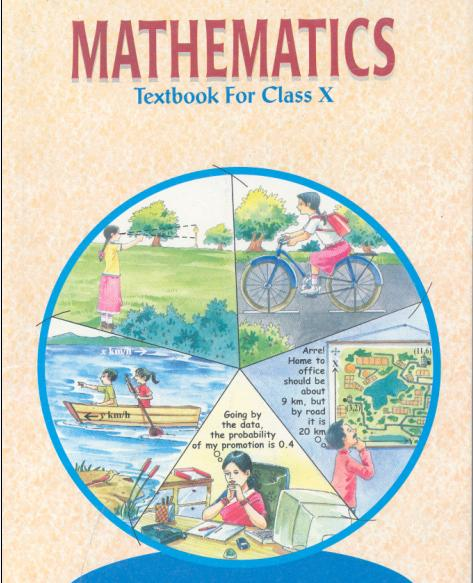## NCERT Solutions for Class 10 Maths Circles

###### 1. How many tangents can a circle have?

Ans. A circle can have infinitely many tangents since there are infinitely many points on the circumference of the circle and at each point of it, it has a unique tangent.

NCERT Solutions for Class 10 Maths Exercise 10.1

###### 2. Fill in the blanks:

(i) A tangent to a circle intersects it in _______________ point(s).

(ii) A line intersecting a circle in two points is called a _______________.

(iii) A circle can have _______________ parallel tangents at the most.

(iv) The common point of a tangent to a circle and the circle is called _______________.

Ans. (i) A tangent to a circle intersects it in one point.

(ii) A line intersecting a circle in two points is called a secant.

(iii) A circle can have two parallel tangents at the most.

(iv) The common point of a tangent to a circle and the circle is called point of contact.

NCERT Solutions for Class 10 Maths Exercise 10.1

###### 3. A tangent PQ at a point P of a circle of radius 5 cm meets a line through the centre O at a point Q so that OQ = 12 cm. Length PQ is:

(A) 12 cm

(B) 13 cm

(C) 8.5 cm

(D)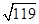cm

Ans. (D)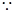PQ is the tangent and OP is the radius through the point of contact.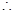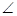OPQ =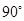[The tangent at any point of a circle is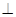to the radius through the point of contact]In right triangle OPQ,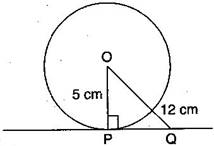OQ2 = OP2 + PQ2 [By Pythagoras theorem]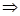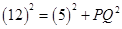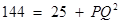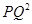= 144 – 25 = 119PQ =cm

NCERT Solutions for Class 10 Maths Exercise 10.1

###### 4. Draw a circle and two lines parallel to a given line such that one is a tangent and the other, a secant to the circle.

Ans.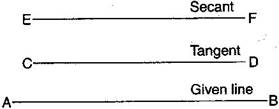## NCERT Solutions for Class 10 Maths Exercise 10.1

NCERT Solutions Class 10 Maths PDF (Download) Free from myCBSEguide app and myCBSEguide website. Ncert solution class 10 Maths includes text book solutions from Mathematics Book. NCERT Solutions for CBSE Class 10 Maths have total 15 chapters. 10 Maths NCERT Solutions in PDF for free Download on our website. Ncert Maths class 10 solutions PDF and Maths ncert class 10 PDF solutions with latest modifications and as per the latest CBSE syllabus are only available in myCBSEguide.

## CBSE app for Class 10

To download NCERT Solutions for Class 10 Maths, Computer Science, Home Science,Hindi ,English, Social Science do check myCBSEguide app or website. myCBSEguide provides sample papers with solution, test papers for chapter-wise practice, NCERT solutions, NCERT Exemplar solutions, quick revision notes for ready reference, CBSE guess papers and CBSE important question papers. Sample Paper all are made available through the best app for CBSE### 6 thoughts on “NCERT Solutions for Class 10 Maths Exercise 10.1”

1. Thanks

2. Nice

3. Thanks

4. Thanks

5. Thankyou

6. i like this apps